László Mester
The new physical-mechanical theory of
granular materials
2009
- 2 -
Contents
Introduction ………………………………………………………………………….3
Granular material as a distinct state of matter …….…………………………………4
Physical properties of the granular material in relation to
the different states of matter …………………………………………….…..6
Granular material as a state of matter …….………..…………………………10
Physical-mechanical basic laws of the non-cohesive granular materials …………..14
Law I ………………………………………………………………………….15
Law II ………………………………………………………………………...15
Law III ………………………………………………………………………..17
Law IV ………………………………………………………………………..24
Stresses in the non-cohesive granular materials ……………………………………26
Active stress state …………………………………………………………………..31
Development of the active stress state ………………………………………..31
Pressures acting on the vertical retaining wall ……………………………….36
Arch formation of granular materials …………………………………………...…39
Condition of the arch formation ……………………………………………...39
Character of the discharge ……………………………………………………44
Mechanism of the arch formation …………………………………………….46
Geometric equation of the arch ………………………………………………47
Principle of the hopper design ………………………………………………..50
Experimental results ………………………………………………………….53
Stresses in cohesive granular materials …………………………………………….55
Lateral pressure ………………………………………………………………55
Inclination angle of the free slope ……………………………………………59
Active stress state …………………………………………………………….64
Summary …………………………………………………………………………...69
Bibliography ……………………………………………………………………….73
- 3 -
Introduction
Following Coulomb’s and later Rankine’s work the physical-mechanical theoretical
research of granular materials has been characterised by the use of stress analysis
deduced for solids since the 18th century. Others seem to detect the characteristic
features of viscous liquids in granular materials, therefore they describe the physical
behaviour of granular materials using the laws pertinent to viscous fluids. In my
opinion most of the theorems, which were put for cotinuums, cannot be applied to
the aggregation of separate, solid granules. Only those natural laws can be
considered as the starting point of examination, which are also valid for the universal
material.
This work breaking away from the previous tradition would like to approach the
physical mechanical properties of granular materials from a new point of view. As a
result, the critical analysis of theories formulated earlier in this research area is not
the objective of this paper, since the new principles were laid down irrespective of
those hypotheses. Contrary to the continuum theory, by examining the equilibrium
and kinetic state of individual granular particles this new thesis is based on simple
experiments, on the Newtonian laws, and on an empirical law, the law of friction.
- 4 -
Granular material as a distinct state of matter
The physical appearance of materials found in nature is quite varied. The most
substantial part of the Earth’s surface is covered by oceans, seas, lakes, that is to say,
covered by water. The dry land is more diverse: one can find rocky mountain ridges,
surfaces covered by gentle slopes and deserts with sand dunes. In places the earth is
covered by snow or ice in winter. Above the surface level the wind is blowing, or we
can experience a period of calm, that is to say we can feel the air. The sun is shining
above us and we know that inside the sun one would find another state of matter.
The outward form of the water that covers substantial part of the Earth is in itself
diverse. At normal temperature and pressure water is liquid, but with the increase of
temperature it evaporates more and more quickly, and it turns into water vapour. Fog
or clouds form. When water vapour freezes, and precipitates in cold, then snow falls,
and it condenses into a granular material. When snow melts, the result is liquid,
which in turn becomes solid when it freezes. That is to say water can exist in liquid,
vapour (gas), snow (granular) and ice (solid) states. In each of its phases water has
different physical properties, and behaves conforming to different laws.
Physics differentiates among the most prominent forms of appearance of material by
classifying them into the different states of matter: plasma, gas, liquid and solid
Some material cannot be put strictly under one category, because they bear the
physical properties of two or more states of matter. These materials, however, can be
described by applying the laws pertaining to materials in a similar state of matter.
- 5 -
Granular material cannot be put under any one of the above mentioned four
categories. Furthermore the physical-mechanical properties of granular material do
not make it possible to describe its behaviour successfully using the physical laws of
one or more phases.
A granular material is a conglomeration of large number of solid particles related to
one another, where the granules as the constituent of the aggregate in spite of the
affecting forces retain their form, and the incidentally arising cohesive force between
the granules is substantially smaller than the inner cohesion of the individual
granules.
The overall, coherent physical system of granular material has not been set up yet,
scientific analysis is available only for a few prominent, primarily soil mechanical
problems. Several theories have been applied for the handling of these problems,
which speculations, however led to contradictory results. Furthermore no connection
resting on firm, uniform physical foundations exists between the theories, or if there
is a relation, it is disputable. The majority of mechanical theories dealing with
granular materials apply the method of stress analysis deduced for solids, which
procedure presupposes, that the granular material is a solid phase continuum.
There are theories, according to which granular materials can be approached with
the laws pertaining to viscous liquids, since granular material exhibits viscoelastic
and viscoplastic properties. Although, the difficult theoretical notions provide an
approximate solution to individual mechanical problems, they cannot be applied to
an overall, reliable description of granular material behaviour.
The opinions concerning the state of matter of granular materials are not unanimous.
This is reflected in the fact that granular material does not have a single uniform
name, for example the following designations: scattering, powderlike, loose,
granulated, grainy, particulate, granular and gritty are all used.
The physical behaviour and properties of granular materials exhibit substantial
qualitative difference from the materials in other states of matter, and should
therefore be considered an additional state of matter in its own right
- 6 -
The idealised notion of granular material makes the simplified explanation of its
physical behaviour, properties and origin possible, similarly to the hypotheses
applied to perfect gases, ideal liquids and crystalline solids. The ideal granular
material is a conglomeration of large number of solids (granules) where the mass of
the solid particles is small compared to the mass of the material, in this aggregate
attractive force does not operate between the particles, Coulomb friction rule
governs them.
Physical properties of the granular material in relation to the different
states of matter
The basis for classification according to states of matter depend on the question
whether the material can hold its own shape and volume or not. The basic criteria of
classification of the three classical states of matter are the following:
- gases: have no definite shape or volume;
- liquids: have no definite shape, but have definite volume
- solids: have definite shape and volume.
Regarding the question of definite shape and volume it is the characteristic of the
granular material that:
- In part it has definite shape, the granular aggregate holds its shape in the angle
of repose, but under this angle it takes the shape of its container. This attributive
places the material between liquids and solids.
- In part it has definite volume, but it can be compressed to a limited extent. The
compressibility of granular materials stands between the compressibility of gases
and solids.
The researches on substance structure concerning the states of matter found that the
determining factors in the question whether a material holds a definite shape or
volume lie in the physical properties of its constituents and the nature of interaction
between these particles. For this reason modern physics studies the kinetic state of
the smallest particles attributed to the material, their relative position and the particle
interaction when defining the different states of matter. This made it possible that in
- 7 -
natural science, in addition to the three classical states of matter, a fourth state of
matter, was accepted, the plasma state.
It seems to be necessary to emphasise the expression smallest constituent
characteristic of the material, since it is of primary importance in the definition of
the different states of matter. Consequently
- A material in plasma state consists of the disintegrated parts of molecules or
atoms, the molecule ions or atomic ions. The plasma state is characterized by the
interactions of atomic or molecule ions and electrons, and not by the other parts of
the atom or molecule.
- The physical properties of a material in the gas state are determined by the
interactions of gas molecules in case of noble gases the interactions of atoms.
- Regarding liquids the determining factors are again the movements of the
atoms or molecules, and the nature of relation between them. In the case of water it
is the interactions of H
2
O molecules and not the hydrogen or oxygen atoms, or the
water drop, which characterize the liquid.
- In the case of crystalline solids the physical properties of a material in the solid
state can be explained with the nature of interaction of the atoms, molecules or ions
positioned in the lattice nodes of a crystal structure, and cannot be described for
example with the interactions of elemental crystals and crystallites, or with the
individual properties of the atoms and its parts, which constitute the molecules
positioned in the lattice points.
- In the case of granular material the smallest constituent characteristic of the
material is the granule. The atomic particles, the atoms and molecules that constitute
the granules, are not direct characteristics at least in physics they cannot be
regarded as significant physical properties of a material, just like as in the case of
gases and liquids, where material is not characterised by the atomic particles, which
constitute the atom or molecules, or by the individual physical properties and
interactions of atoms either.
The most significant characteristics of the three classical states of matter can be
summarised in the following way:
- 8 -
Gaseous state: The molecules of gases in case of noble gases the gas atoms
move freely in the space available for them, they collide elastically with one another
in a random motion. The average distance between the constituting particles of gases
is relatively big in proportion to their size, the intermolecular forces between the
particles are very weak. In the case of ideal gases intermolecular force can be
disregarded. Molecules move with a translational, rotational and vibrational motion.
Gases evenly fill the space available for them, that is to say they have no definite
shape or volume.
Liquid state: The intermolecular forces between the smallest constituents
characteristic of liquids, between the atoms or molecules are strong enough to
prevent the particles moving away from each other as a consequence of thermal
motion, but not strong enough to prevent their change of position. Compared to
gases, the translational motion of the molecules are smaller, while they also carry
out rotational and vibrational movement. Due to their motion and proximity the
constituting particles collide elastically with one another all the time, thus touch one
another, therefore liquids have a definite volume. The force of attraction between the
particles is so small compared to the Earth’s gravitational force that it is not enough
for individual shape formation, as a result liquids have no definite shape.
Solid state: The smallest constituting particles, characteristic of solids are the atoms,
molecules or ions. Their position is fixed and geometrically determined in a
crystalline structure, particles carry out only vibrational motion. The intermolecular
forces are strong, which prevent their permanent displacement from their state of
equilibrium. As a result solids have definite shape and volume.
Granular material exhibits significant differences from the aggregational properties
of the three classical states of matter. The constituents of an ideal granular material,
the granules are at a relative rest. There are no forces of attraction between the
particles, the material is kept in an aggregate by the compressive forces originating
from the gravitational force, by the shear forces arising on the surface of the
granules, and by the static friction force. Due to these forces the ideal granular
material remains stable until the angle of repose is reach, thus it has only partly a
definite shape. The constituting particles are in constant contact, therefore in
- 9 -
quiescent state granular material has definite volume. Under pressure the material is
compressed, the granules take up a more efficient space filling position. The
compressibility of granular material is small compared to gases, but it is big in
comparison to solids.
The physical properties of ideal granular material exhibit the following significant
differences in characteristics compared to the features of other states of matter:
- In contrast to gaseous state: the constituting particles are in constant contact
with each other, and it has definite volume;
- In contrast to liquid state: granular material has in part a definite shape;
- In contrast to gaseous and liquid state: The constituting particles are in a
relative collision free, quiescent state and static friction force shear force arise in
them;
- Solid state: there is no attractive force between the constituting particles,
therefore granular material has only in part a definite shape.
Granular material exhibits such qualitative differences concerning the most
substantive characteristics of the different states of matter that its definition as a
separate state of matter in its own right becomes justified.
The brief, straight to the point definition with no pretence to completeness of the
idealised case of the states of matter is the following.
Perfect gas: disordered aggregate of molecules (in case of noble gases atoms) with
no intermolecular forces, where the molecules move far apart from each other,
undergoing random elastic collisions.
Ideal liquid: Aggregate of molecules moving close to each other, undergoing
constant elastic collisions.
Crystalline solid: The ordered aggregate of vibrating atoms, molecules or ions,
which are fixed in their structure with great force.
Ideal granular materials: the aggregate of relatively static particles, which are in
constant contact with each other, in this assembly the force between the constituting
particles is composed of the compressive force arising from the gravitational force
and of the friction force, which is proportional to it, there is no cohesion force
between the particles.
- 10 -
The notion of granular material, as a separate state of matter is primarily important
from a mechanical viewpoint. (The basis for categorization into different states of
matter has a mechanical origin: the reason for having a definite shape or volume can
be deduced from the intermolecular forces between the constituting particles.) The
mechanical properties of the different states of matter show distinctive, substantial
differences:
- gases respond to an increase in pressure with the significant reduction of their
volume (at constant temperature the multiplication product of volume and pressure
is constant), therefore they can withstand compressive stress only in part, at the
expense of volume change. In perfect gases there is no attractive force between the
constituting particles, therefore no tensile stress can arise in the material. In static
not flowing gases no shear stress arises.
- liquids has small compressibility, from a mechanical point of view they can be
regarded incompressible, therefore they can withstand compressive stress. The
intermolecular forces are strong enough to prevent the constantly colliding
molecules from moving far away from one another. The state of equilibrium or
stability can only be attained under a given outside pressure, that is to say, from a
mechanical standpoint a liquid cannot withstand tensile stresses. (When the pressure
is around p=0 the liquid breaks up, its molecules fly apart and turn into gaseous
state) There is no friction in ideal liquids, in real liquids static friction does not arise
either.
- solids can be regarded as incompressible, the constituting particles join
together with great force, therefore they can withstand tensile, compressive and
shear stress.
- ideal granular material has small compressibility, therefore it can withstand
compressive stress. In the non-cohesive granular materials only shear stress arises in
addition to compressive stress, no tensile stress manifests itself.
Granular material as a state of matter
The notion that granular material must be regarded as a separate state of matter can
be justified not only because its distinct physical properties, which differentiate it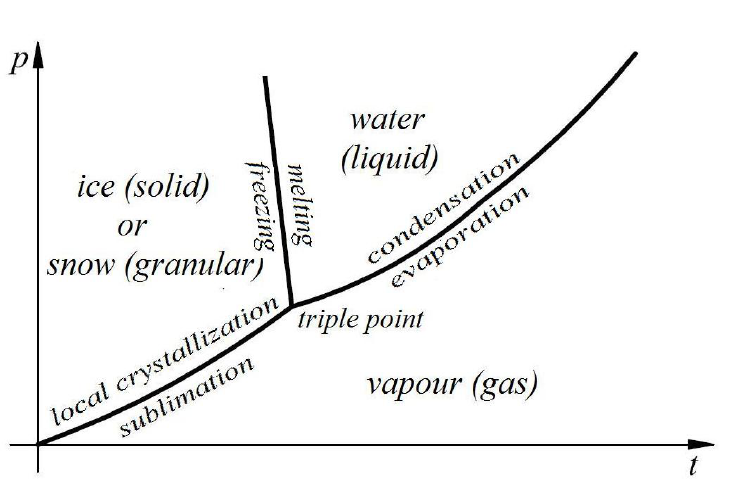- 11 -
from other states of matter, but also because granular material is one of the existing
outward forms of raw material, that is to say it is one of defined states of matter.
Granular material as the conglomeration of large number of solids, where the
constituting solids are small in proportion to the total mass of the material
generally comes into being when large-sized solids are mechanically cut up, or when
the solids themselves break up into smaller pieces. Its formation, that is to say, the
bringing of the material into a granular state can be achieved not only in a
mechanical way, as it is also true for the granular materials in nature, which were
formed not exclusively by mechanical disintegration either. Granular material can be
produced via a thermodynamic method.
It is known, that if the kinetic energy of the molecules of a liquid exceeds a
threshold it changes into gaseous state and if it goes below another threshold the
liquid turns into solid and the process of crystallization begins. The threshold values
of the thermodynamic state parameters characteristic of the different states of matter
can be illustrated in a p t (pressure-temperature) diagram. In Figure 1 the p t
diagram of H
2
O can be seen.
Figure 1. p-t diagram of H
2
O
- 12 -
The curve that joins the triple point and the origin of the p t diagram is called the
sublimation curve. The material passes from the solid ice phase into the vapour
phase of the gas state by for example pressure reduction and by the crossing of the
sublimation curve. Crossing the sublimation curve backwards, from vapour phase
granular snow is formed not ice. The granular material in case of water, the snow
comes into being as a result of crystallization in the local clusters. The process can
be called local crystallization, the physical explanation of which lies in the
phenomenon that the molecules which move with slow translational motion (under
low temperatures) cannot leave the attraction field of the van der Waals type forces
for example as a result of heat loss therefore the translational motion of the
molecules ceases.
The molecule pair - bonding the new molecules, which collide into them - form a
crystal lattice, the growing crystals then bring about the granules. The density of
molecules in the gaseous state is very low in comparison to the molecular density of
the solid state, therefore the local crystallization processes, which are relatively far
from one another bring about the multitude of separate granules, which after having
precipitated form a granular conglomeration.
Under constant temperature the process of getting from gas phase to granular phase
is accompanied by heat loss, which is the sum of the melting and the evaporation
heat.
The states of matter change at the phase boundaries of the p t diagram. If the
matter crosses the sublimation curve from the solid phase toward the gas phase, we
will get a gas, however changing the direction crossing the curve from the gas phase
we will obtain a granular material. Thus the states of matter, in compliance with the
direction of crossing the phase boundaries are the following:
gas local crystallization granular melting liquid evaporation gas;
nevertheless, from the other direction:
gas condensation liquid → freezing solid → sublimation gas.
From granular phase to solid phase we can get by crossing the same phase boundary
twice:
- 13 -
granular melting liquid → freezing solid.
Granular material can be produced directly from liquid phase if we place a multitude
of crystal nuclei - which are approximately at an equal distance from one another -
into a supercooled liquid at the same time. The crystal growth is hindered by the
neighbouring crystals, whose geometric crystal position is not symmetrical or
congruent, therefore no or only occasional lattice forces develop between the
crystals, the inner cohesive force of the individual granules are substantially greater
than the incidental cohesive forces acting between the granules.
The substantial physical properties of the granular material differ significantly from
the characteristics of those materials whose chemical properties are identical,
nevertheless belong to the solid, liquid or gaseous state. Its volume weight, its
refractional, thermodynamical, acoustic, electric and mechanical properties and
behaviour, and the fact that most material can be brought into granular state justifies
the classification of the granular material as a distinct state of matter by its own
right.- 14 -
Basic physical-mechanical laws of the non-
cohesive granular materials
I. In the non-cohesive granular materials only compressive and shear stresses
can develop.
II. In the non-cohesive granular materials at a quiescent state the stresses
developed by the vertical-direction compressive stresses act downwards in the
0
90
zone measured from the vertical direction. (
is the angle of friction of
the material.)
III. The value of the lateral pressure rising from the self-weight of the non-
cohesive granular material is (
2
h
), the half of the product of the depth (h) and
volume weight (γ), its direction deviates from the horizontal downwards with the
angle of friction developed in the material, if the surface is horizontal and over the
given depth the material fills the space evenly closing an angle
with the
horizontal.
IV. The non-cohesive granular materials conform to the physical-mechanical
laws characteristic of them until their constituting elements, the grains keep their
relative quiescent state. When the grains go into motion collide with each other -,
the granular materials behave according to the physical-mechanical laws of the
liquids.
The physical-mechanical laws of the non-cohesive granular materials prevail with a
statistical character, because the material itself consists of a multitude of different
grains.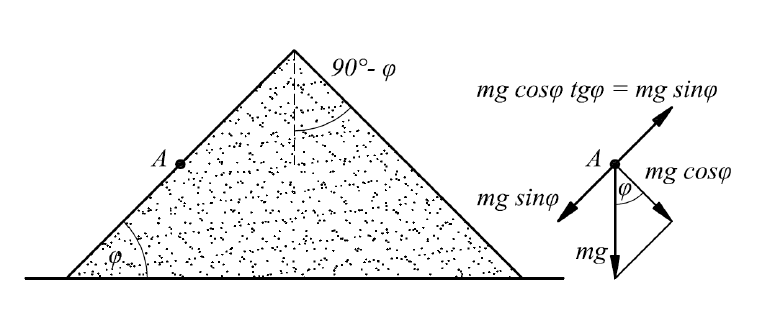- 15 -
Law I
Law I is the physical-mechanical definition of the non-cohesive granular materials.
In ideal liquids only compressive stresses develop, the non-cohesive granular
materials are capable of withstanding compressive and shear stresses, while solids
are capable of bearing compressive, shear and tensile stresses. The non-cohesive
granular materials differs from the solids in the respect that they are not capable of
withstanding tensile stress, and they are distinct from the ideal liquids because shear
stresses also develop in them. At the same time the components of the liquids are in
constant relative motion collide with each other -, while the components of the
granular materials, the grains are in a relative quiescent state.
Law II
Law II formulates the direction of the spreading of the vertical compressive stresses.
The natural stability of the free slope provides its experimental proof (Figure 2).
Figure 2. The boundary equilibrium position of the grain located
on the side of the slope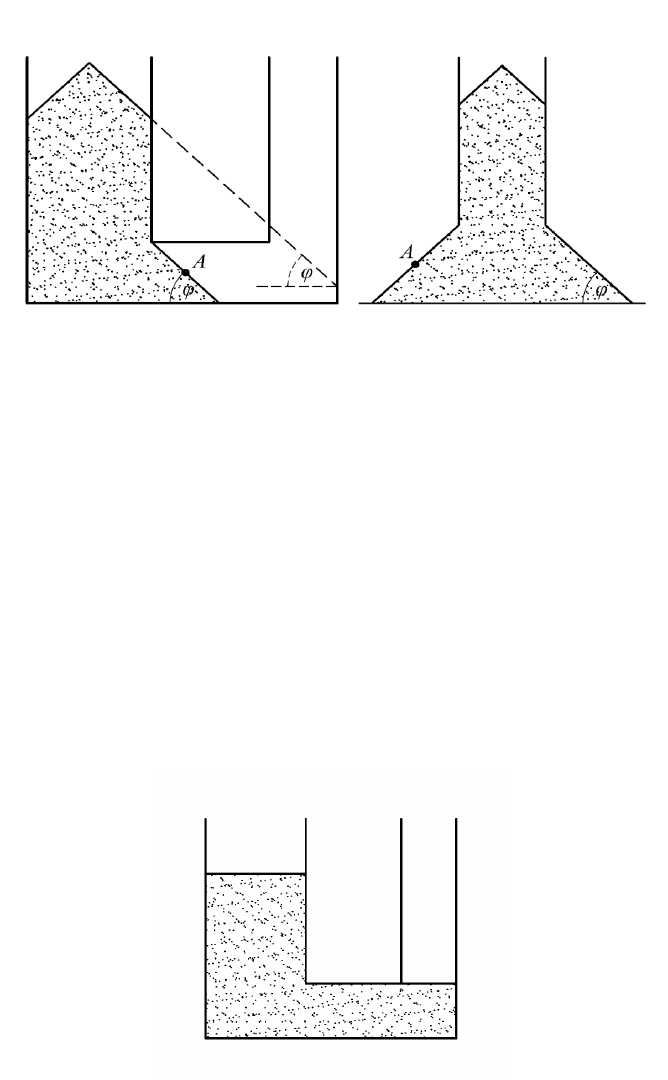- 16 -
In the conglomeration of grains the self-weight of the grains produces the vertical
compressive stress. If a stress vector acted on the grain marked A located on the side
of the slope in boundary equilibrium position - inclined at an angle of more than
from the vertical then the grain would loose its equilibrium position and
slide down.
Figure 3. The stresses developed by the vertical compressive stresses
incline at an angle bigger than
to the horizontal.
Further experiments prove the correctness of the Law II (Figure 3). If a compressive
stress making a smaller than
angle with the horizontal acted on the grain marked
A, the angle of inclination angle
of the natural slope would be smaller than
. If
the vertical compressive stress induced, for example, a horizontal stress, it would
thrust down the grains located on the side of the slope. The material would spread
and would take a kind of shape that is illustrated in Figure 4. However, it does not
exist.
Figure 4. If the compressive stresses induced horizontal stresses, this
would be the position of the granular material.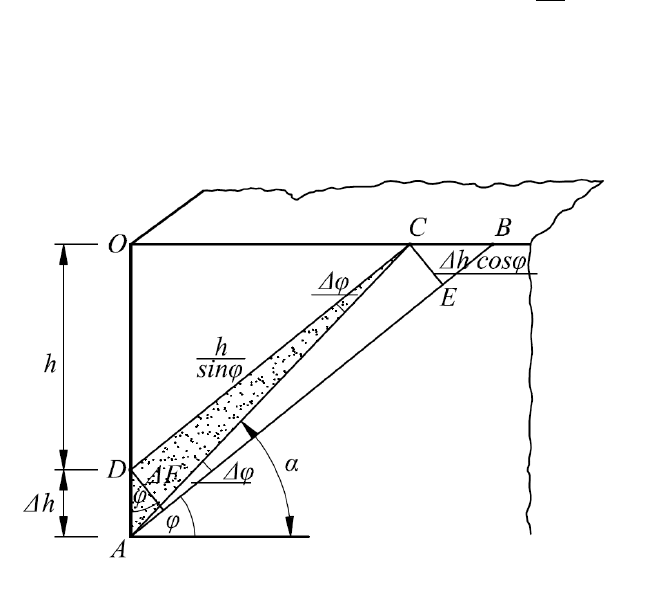- 17 -
Law III
In the cases described in the Law III the lateral pressure is
2
h
, and its direction
inclines from the horizontal downward with the angle of friction rising in the inside
of the material. Its proof is as follows:
Figure 5. Infinite Quadrant of the Horizontal Terrain
Figure 5 shows that part of the non-cohesive granular material aggregate of infinite-
expansion and horizontal-terrain, which is cut out theoretically by two vertical
planes perpendicular to each other, (consequently, it shows an infinite quadrant of
the horizontal terrain,) which makes the planar execution of the mechanical tests
possible. According to the Law II, from the material part under the section AB only
reaction stresses produced by the material part over the plane AB can act on the
plane OA. If we took off the granular material located in the triangle OAB, then the
material would remain stable in the natural angle of repose AB inclining at an angle
to the horizontal. On the plane OA, stresses can only rise from the self-weight of
the granular material located in the space part OAB. On the plane AB an equilibrium
boundary position exists; the material having a friction coefficient of
tg
does
not slide as yet on the slope with an inclination of angle
. If we increased the slope
angle with
of a very low value, then the material above it could slide down with- 18 -
constant acceleration on the slope with the inclination angle
, i.e. it would
exert a force in direct proportion to its mass and acceleration on the plane OA. Due
to the physical properties of the granular materials there are infinitely many slopes
with an inclination angle
, above which angle, on the slopes increased by the
angle
, the weight of the materials exerts slope-direction stresses on the plane
AO. According to Figure 5, on the slope with an inclination angle of
,
produced as a result of the depth increased by Δh, the granular material ADC weighs
heavily on the slope with its self-weight (ΔG) and with the weight of the material
located above it (G). The material amount ADC is supported on section Δh. The
projection of the surface section Δh, perpendicular to the slope is
)cos(
hF
. Considering that the Δh is very little, therefore, the stress
distribution can be considered even, so it can be stated for the slope-direction stress
developed there:
,
)cos)(sin(
F
GG
In the unit-length space part
,
2
2
ctg
h
G
and
,cos
sin2
1
h
h
G
that is,
,
2
ctgh
h
G
furthermore,
).cos(
hF
The (
cossin
) in the equation can also be expressed:
)cos()sin(
tg
,
),sinsincos(cos
cos
sin
sincoscossin
,sin
cos
sin
sincos
2- 19 -
)sin(cos
cos
sin
22
,
.
cos
sin
Substituting the values of the G, ΔG, ΔF and
cossin
into the relation
written for the
:
)cos(
cos
s in
s in
cos
22
2
h
hh
ctg
h
,
,
)cos(
cos
sin
)(
2
h
hhctg
h
,
)sinsincos(cos
cos
sin
)(
sin
cos
2
h
hh
h
,
s in
s in
cos
coss in
2
h
hhh
,
sin
cossin
2
2
h
tg
h
hhh
but the
tg
can be expressed from the triangle ACE of Figure 5:
,
sin
sin
cos
h
h
h
tg
,
sin
cossin
2
hh
h
tg
therefore,
,
sin
sin
cossin
cossin
2
2
2
h
hh
h
h
hhh
,
sinsin
2
22
hhh
hhh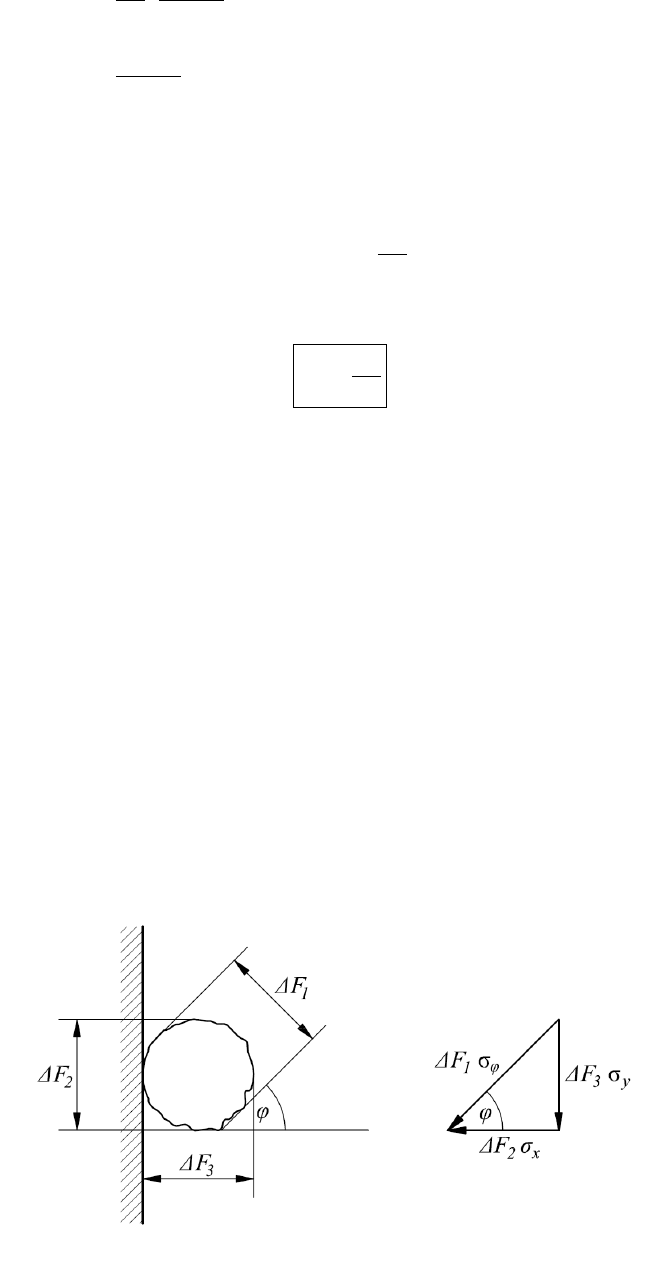- 20 -
,
2 h
hhh
,
2
hh
if the Δh is very little, that is Δh 0, then α
, consequently
, i.e. the
direction of the stress inclines at an angle
to the horizontal. Consequently,
,
2
lim
0
h
h
that is,
2
h
.
As a result of the deduction it can be established that the stress distribution is linear
against the depth.
However, in case of granular materials one cannot speak of a stress in the classical
sense, since the force effects are transmitted at the contact points of the granules, i.e.
from one point to another, not on a surface perpendicular to the given direction. Not
on a surface, because the material is a discontinuum and the grains touch each other
only at points. Therefore, the meaning of the stress can be interpreted as the average
force imparted to one surface and these average forces are transferred from one
granule to another. The direction and size of these forces manifest themselves as a
statistical average on a given surface.
Figure 6. Division of the average force acting on the grain located
next to the wall into a horizontal and a vertical component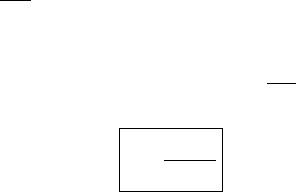- 21 -
Let us examine what the magnitude of the force is, which the above deduced stress
, inclining at an angle
to the horizontal, exerts on a vertical wall. Through the
contact points force arrives from the neighbouring granules at the grain which is
supported by a frictionless vertical wall shown in Figure 6. The resultant of this
force effect should be equal with the product of the stress
calculated for the
surface
1
F
, i.e. considering its magnitude and direction the compression force
acting on this grain corresponds to the statistical average of the force exerted at this
depth in the given granular material. This granule presses the vertical frictionless
wall with a horizontal
x
F
2
force on the wall section
2
F
at the contact point. The
vertical-direction force
3
F
y
is received by the grain or grains located under it.
The surface of sections
1
F
,
2
F
and
3
F
are equal surfaces on statistical average,
because, considering their shape and position, the grains are spheres on statistical
average; the projections of the spheres from any direction are of equal surface area.
(If a granular material for example, rice consists of oblong grains; considering
the random arrangement of the grains the average of their projection taken in any
direction is a circle, i.e. the grains must be considered as spheres on statistical
average.)
Consequently, it can be written for the vector triangle of the Figure 6
cos
12
FF
x
,
but since
21
FF
,
therefore
cos
x
.
It comes from the result of the above consideration that in granular material the
stresses the average forces calculated for a given surface can be decomposed or
cos
x
equation the factor of static pressure is
substitutions, that is
h
y
cos
2
h
x
,
so
2
cos
.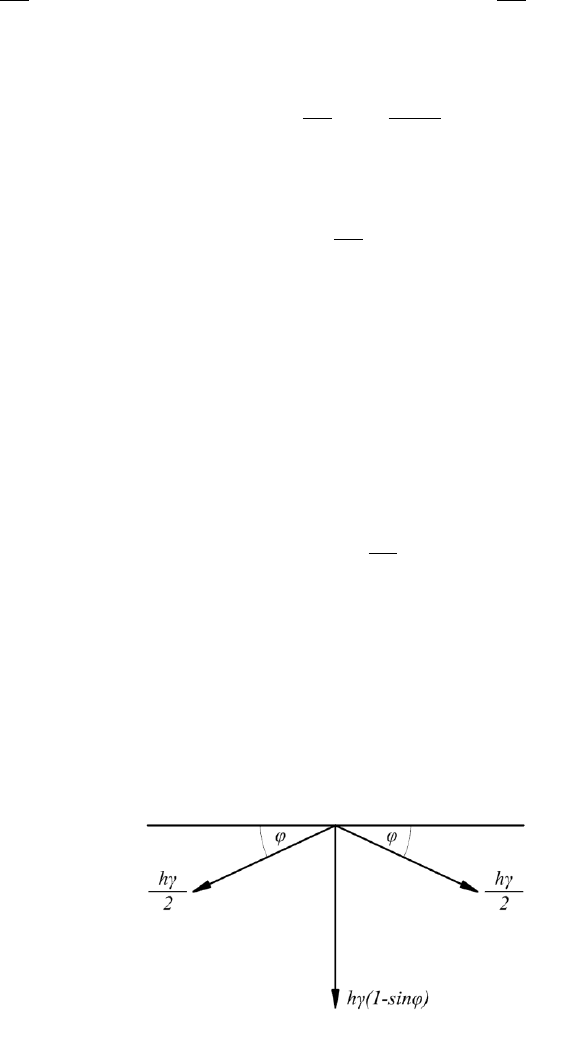- 22 -
Up to this point, only the shear stresses generated by the stresses acting
perpendicularly to the direction inclining at an angle
to the horizontal and rising
from the self-weight were taken into account at the deduction of the static pressure.
The shear stresses
y
, produced by the horizontal stress components -
cos
2
h
x
- reduce the vertical stresses with
tg
h
y
cos
2
,
that is
,
cos
sin
cos
2
h
y
so
sin
2
h
y
.
Considering that the stresses
act in pairs on the theoretic plane OA assumed
inside the granular material (Figure 5), therefore, the vertical-direction stresses
are reduced by 2
y
, consequently
yy
h
2
,
that is
,sin
2
2
h
h
y
and
)sin1(
h
y
.
Consequently, the figure of the stresses acting inside the non-cohesive granular
materials at quiescent state can be constructed (Figure 7).
Figure 7. Stresses acting at a quiescent state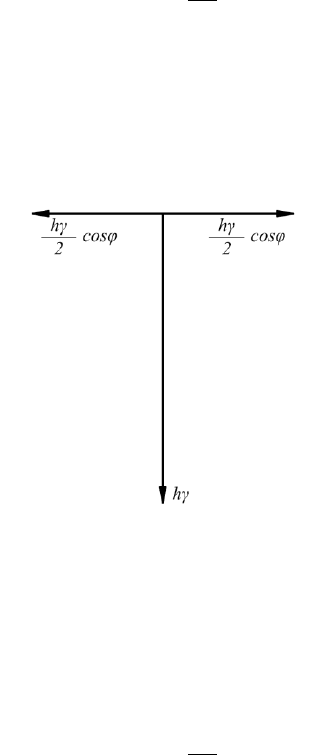- 23 -
If the plane OA according to Figure 5. is a theoretic plane assumed inside the
granular material, then the angle of friction is
there. In this case a compressive
stress of
, inclined at an angle
to the horizontal acts on both sides of the plane
OA (Figure 7.). On the plane OA the
’s can be divided into horizontal and
vertical components (Figure 8.). The horizontal components have the value
cos
2
h
x
,
they are perpendicular to lane OA and satisfy the action-reaction law. The vertical
stress components of
y
complement the vertical stresses to symmetrically to
plane OA in a reciprocal way.
Figure 8. The horizontal and vertical stress component at a quiescent state
If the plane OA according to Figure 5 is a frictionless wall, then that is capable of
taking only horizontal stress, i.e. the horizontal component of the
, which is
cos
2
h
x
.
At the same time the vertical component of the
complements the vertical stress
component of the material OAB to .
If the plane OA is an actual rough, rigid wall, which serves to support the OAB
material amount, then the development of the static pressure can be interpreted as
follows.- 24 -
After filling up the OAB material amount behind the OA wall the value of the
2
h
is not reached immediately, since due to the inclined stress effect and
because of the friction rising on the wall a weight force intake is realised on the
plane OA. As a result of the weight-force intake the force acting on the plane AB is
reduced; so it is not capable of producing stresses with the value of
2
h
and in the
direction AB. The freshly filled-in material comes to a standstill by finishing its
consolidation motion. As a result of the quiescent state the friction rising on the wall
is reduced to zero, consequently the stresses
are decomposed into their
horizontal and vertical components. In the OAB material the vertical components
complement the vertical stress components to , and supplement the weight of the
OAB material to
ctg
h
2
2
. At this time the horizontal component of stress
acts
on the OA wall. Consequently the horizontal component of the static pressure is:
,cos
2
h
x
and, therefore the factor of static pressure is (λ):
2
cos
.
Law IV
Law IV can be proved experimentally.
As a result of the experiment demonstrated in Figure 9, due to the collision of the
grains, the granular material behaves according to the laws of the communicating
vessels.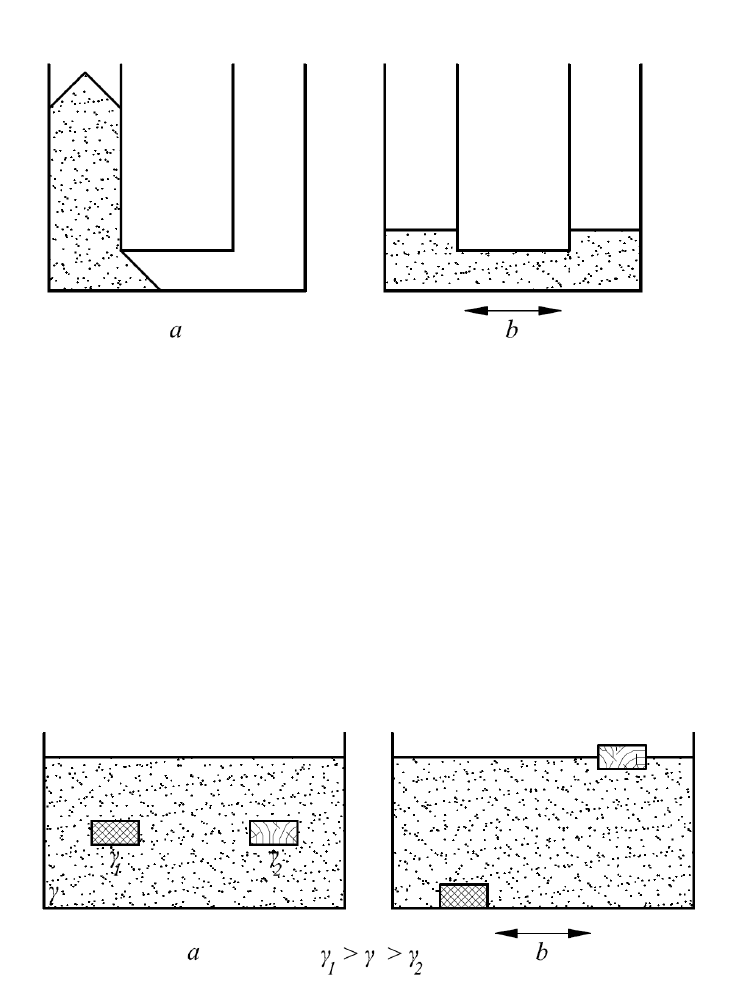- 25 -
Figure 9. a) state of rest b) state formed as a result of vibration
In Figure 10 as a result of the vibration, and due to the collision of the grains the
body with bigger volume weight γ
1
and the body with smaller volume weight γ
2
, -
which were place into the granular material with volume weight γ - sinks to the
bottom of the vessel, or floats on the surface of the granular material respectively;
consequently the law of Archimedes prevails.
Figure 10. a) state of rest b) state developed as a result of the vibration
As a result of the vibration the components of the granular material, the grains
collides into each other, and due to this effect the pressure changes to in every
direction.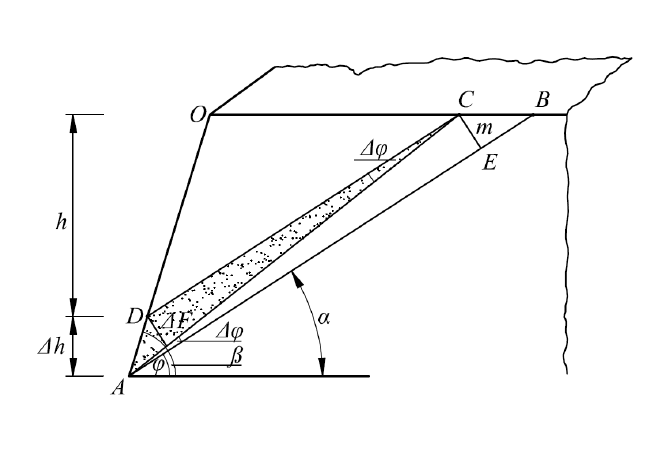- 26 -
Stresses in the non-cohesive granular materials
We performed the examination of the horizontal plane quadrant cut out by the
vertical plane in the proving procedure of the Law III. If this plane inclines towards
the material compared to the vertical, closing an angle β with the horizontal, - and
the terrain is horizontal, then, generalizing the former deduction the magnitude of
lateral pressure of this granular assembly can be determined in the plane of angle β.
Figure 11. Infinite quadrant of the horizontal terrain confined
with an inclined plane
Using the markings of Figure 1, it can be said that the ADC granular material weighs
on the slope with an inclination angle
, produced as the result of the Δh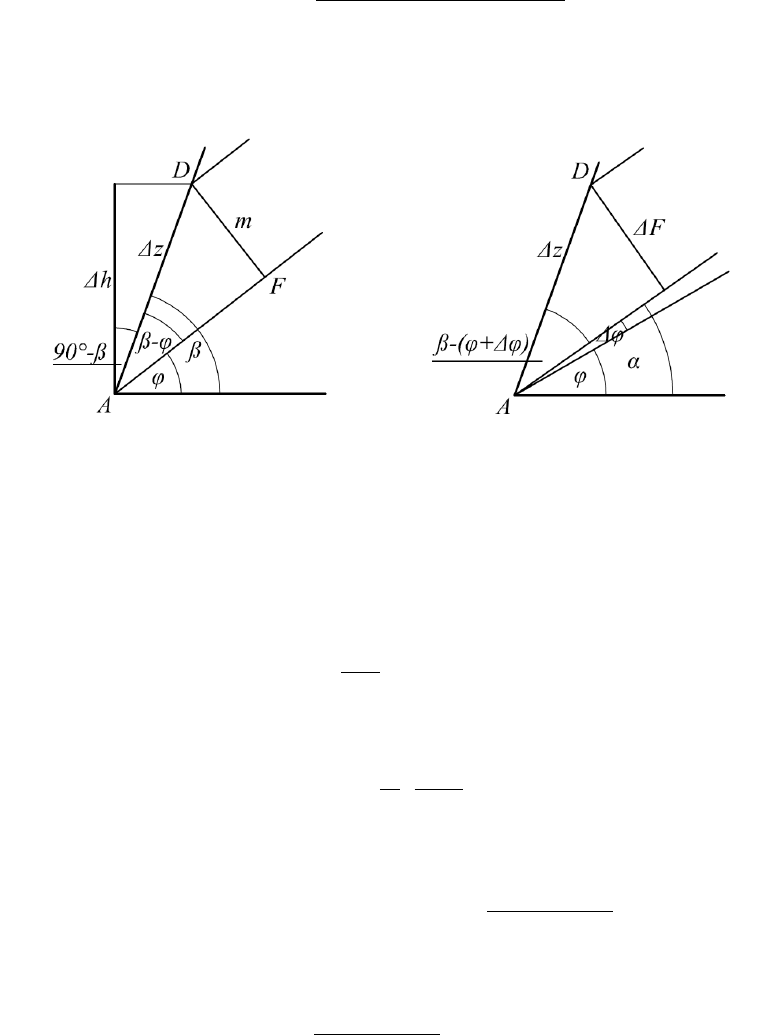- 27 -
depth increase(as it is marked on Figure 11) with its self-weight (ΔG) and with the
weight of the material above it (G). Line segment Δh supports the ADC material
amount in order to prevent its sliding down. Since Δh is very little, therefore, the
stress distribution can be considered even in the area, thus the following can be
formulated for the slope-direction stress rising there:
,
)cos)(sin(
F
GG
where the ΔF is the projection of the surface segment Δh perpendicular to the slope.
Figure 12.
h
part of Figure 11. Figure 13.
F
part of figure 11.
The G given in the equation can be expressed for the unit-long space parts ΔG and
ΔF from Figures 11, 12 and13:
),(
2
2
ctgctg
h
G
and
sin2
hm
G
,
where m can be expressed with the help of Figure 11:
)sin(
zm
, and
,
)90cos(
0
h
z
thus
),sin(
)90cos(
0
h
m- 28 -
that is
),sin(
sin
h
m
Replacing the value of the m into the relation written for the ΔG:
.
sinsin
)sin(
2
hh
G
The value of ΔF can be expressed with the help of Figure 13:
,)(sin
zF
.)(sin
sin
h
F
The equality
cos
sin
cossin
was deduced during the proof of the Law II.
Replacing the value of G, ΔG, ΔF and
cossin
into the relation written for
the σ
α
:
,
cos
sin
)(sin
sin
sins in
)sin(
2
)(
2
2
h
hh
ctgctg
h
,
)(sin
sinsin
)sin(
)(
cos
sinsin
2
hctgctgh
h
h
,
sin)cos(cos)sin(
sin
sincoscossin
)cossin(
cos
sin
2
hctgh
h
h
,
)cos()sin(
)cossin()cossin(
cos
1
2
ctg
ctghctgh
h
h
,
)cos()sin(
cossin
cos2
ctg
ctg
h
hhh
but
ctg
can be expressed from the triangle ACE of Figure 11 by employing the
m
AFCD
m
AE
ctg
,
where:
sin
h
CD
and
)cos(
zAF
,- 29 -
but
sin
)90cos(
0
hh
z
,
therefore
)cos(
sin
h
F
,
so
,
)cos(
sinsin
m
hh
ctg
since
),sin(
sin
h
m
,
)sin(
sin
)cos(
sinsin
h
hh
ctg
)sin(sin
)cos(sinsin
h
hh
ctg
,
);(
)sin(sin
sin
ctg
h
h
ctg
replacing the value of
ctg
into the relation of the
:
,
)cos(
)sin(
)sin()cos(
)sin(sin
)sin(sin
cossin
cos2
h
h
ctg
h
hhh
,
)cos()cos(
sin
sin
cossin
cos2
h
h
ctg
h
hhh
cossin
sin)cossin)((
2
h
ctghhh
,
),1(
2
tgctg
hh
if Δh is very little, that is Δh 0, then α
, consequently
, i.e. the
direction of the stress closes an angle
with the horizontal.
Consequently
),1(
2
lim
0
tgctg
h
h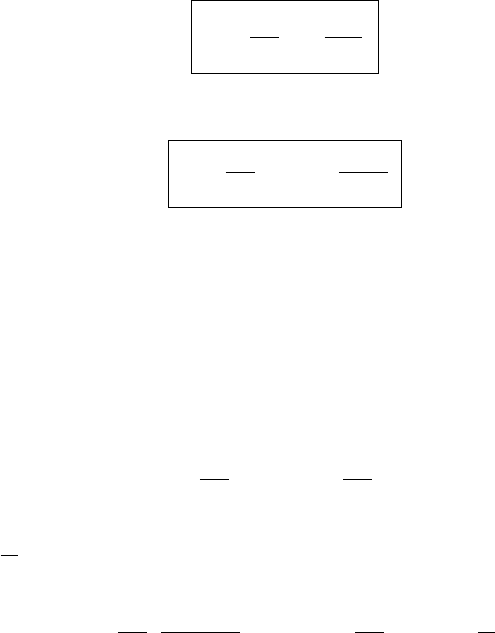- 30 -
that is
tg
tgh
1
2
.
The horizontal component of the
is:
)
sin
(cos
2
tg
h
h
.
Examining the three special values of the slope angle β of the plane, (which is the
angle at which the plane inclines to the horizontal), it can be established that if
, then
0
, i.e. the non-cohesive granular material will stop in the free
slope without support.
If
0
90
, then the static pressure acting on this plane is:
2
h
, and
cos
2
h
h
.
If
2
45
0
, then the static pressure acting on this plane is:
sin1
1
2
h
, and
2
45
2
0
tg
h
h
.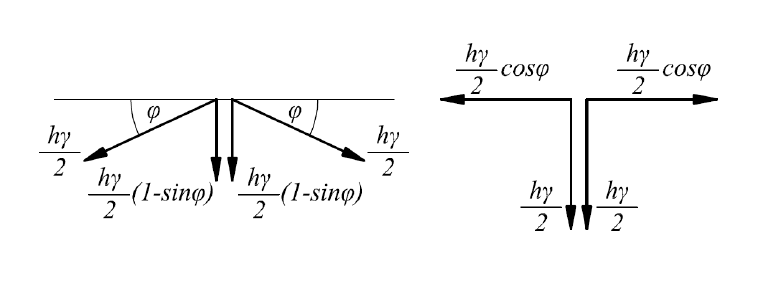- 31 -
Active stress state
Development of the active stress state
The small-size horizontal-direction displacement tilt of the vertical wall
supporting the non-cohesive granular material being in a state of rest causes
expansion in the material. The motion of the material follows the displacement of
the retaining wall into the horizontal direction loosened up, which appears as a
relatively two-direction displacement from a given point of the interior of the
material. As a result of the displacement following the expansion, the effect of the
shear stresses mobilised by the horizontal stress components of the static pressure
ceases (breaks up). The relatively two-direction displacement inside the material
terminates the vertical-direction shear stresses in pairs, therefore the vertical stress
increases to . At the same time the material begins to carry out a consolidation
motion.
Figure 14. Stress model in quiescent a position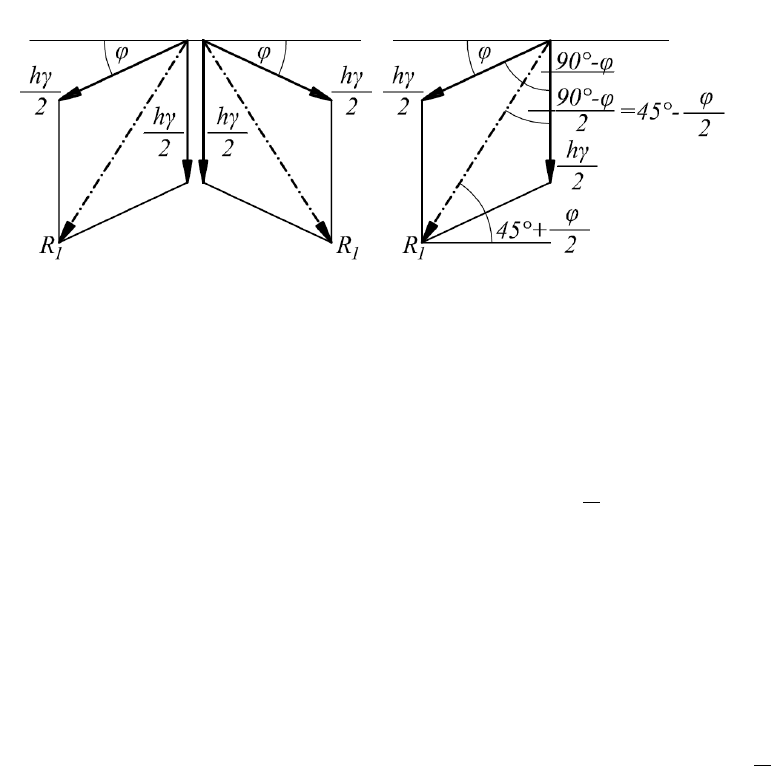- 32 -
The vertical stress of Figure 7 and 8 (state of rest) can be divided into two stresses of
the same size and direction (Fig. 14). The development of the active stress state can
be explained in the horizontal-surface non-cohesive granular materials by the change
of the so obtained stress model. The shear stresses, which were terminated due to the
effect of the expansion, change the stress model of Figure 14 to that of Figure 15.
Figure 15. Change of the stress model in an active state
The consolidation motion occurs in the direction of the biggest stresses, i.e. in the
direction of the stress resultants. The directions of the resultants of the stress pairs
which can be read from Figure 15 incline at an angle
2
45
0
to the horizontal
and at an angle
0
90
to each other. Considering the acting (resultant) stress
directions this stress condition consequently, corresponds to the Rankine active
stress state. Stress
1
R
starts the consolidation motion. This motion is reduced by the
multiplication product of
tg
and stress
2
R
- a stress perpendicular to the stress
1
R
-
,
1
R
mobilises shear stress. The magnitude of stress K inclining at an angle
2
45
0
to the horizontal, consequently is
.
21
tgRRK
Stress
1
R
consists of two stresses.
1
R
and
2
R
can be expressed from the illustrations
of the stress vectors in Figure 16.
Stress
1
R
presents itself as the sum of two stresses; the sum of its stress components
taken for this direction (
2
R
) develops the shear stress.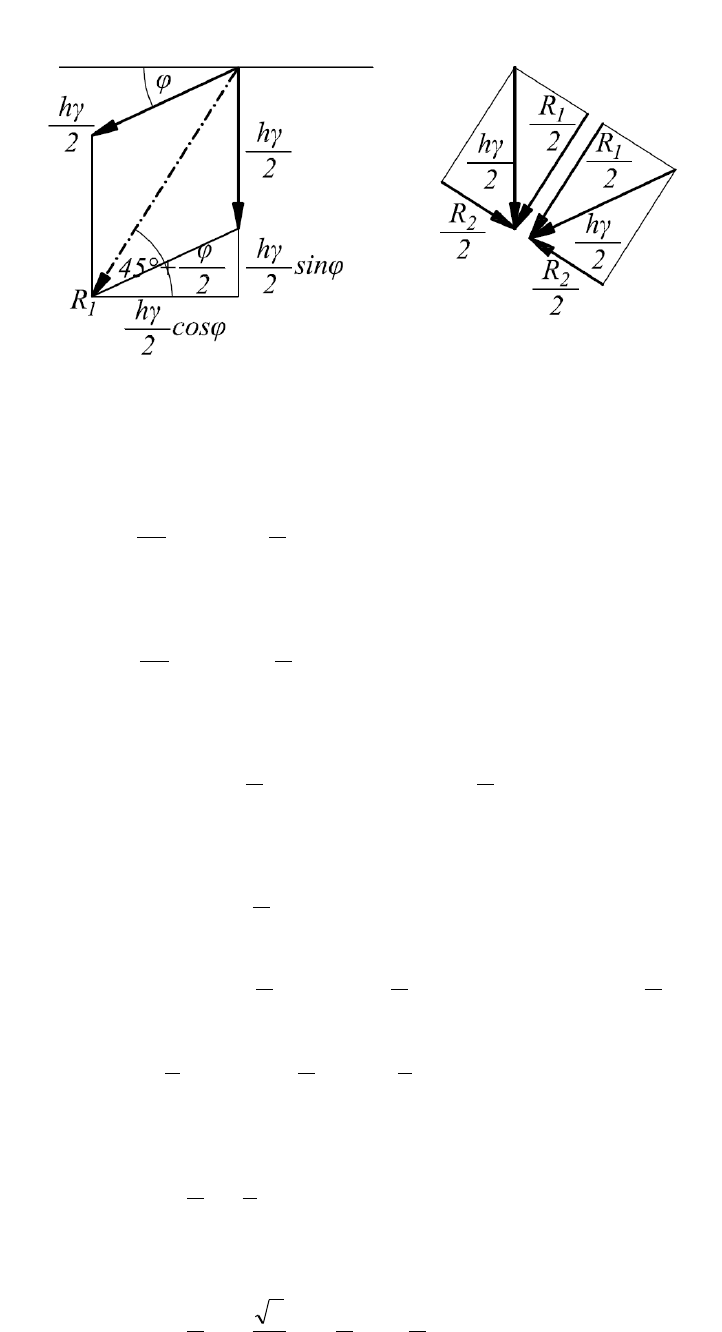- 33 -
Figure 16. The motion started by the resultant stress mobilises shear tress